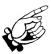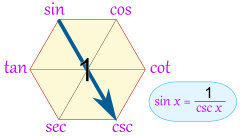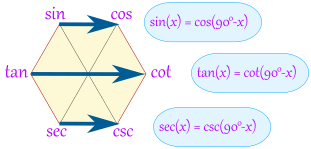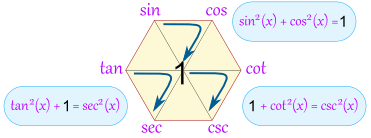# Magic Hexagon for Trig Identities

 This hexagon is a special diagram to help you remember some Trigonometric IdentitiesSketch the diagram when you are struggling with trig identities ... it may help you! Here is how:

## Building It: The Quotient Identities

tan(x) = sin(x) / cos(x)To help you remember think "tsc !"• cot (which is cotangent) on the opposite
side of the hexagon to tan
• csc (which is cosecant) next, and
• sec (which is secant) lastTo help you remember: the "co" functions are all on the right

OK, we have now built our hexagon, what do we get out of it?

Well, we can now follow "around the clock" (either direction) to get all the "Quotient Identities":

 Clockwise tan(x) = sin(x) / cos(x) sin(x) = cos(x) / cot(x) cos(x) = cot(x) / csc(x) cot(x) = csc(x) / sec(x) csc(x) = sec(x) / tan(x) sec(x) = tan(x) / sin(x)
 Counterclockwise cos(x) = sin(x) / tan(x) sin(x) = tan(x) / sec(x) tan(x) = sec(x) / csc(x) sec(x) = csc(x) / cot(x) csc(x) = cot(x) / cos(x) cot(x) = cos(x) / sin(x)

## Product Identities

The hexagon also shows that a function between any two functions is equal to them multiplied together (if they are opposite each other, then the "1" is between them):Example: tan(x)cos(x) = sin(x) Example: tan(x)cot(x) = 1

Some more examples:

• sin(x)csc(x) = 1
• tan(x)csc(x) = sec(x)
• sin(x)sec(x) = tan(x)

## But Wait, There is More!

You can also get the "Reciprocal Identities", by going "through the 1"Here you can see that sin(x) = 1 / csc(x)

Here is the full set:

• sin(x) = 1 / csc(x)
• cos(x) = 1 / sec(x)
• cot(x) = 1 / tan(x)
• csc(x) = 1 / sin(x)
• sec(x) = 1 / cos(x)
• tan(x) = 1 / cot(x)

## Bonus!

AND we also get these:Examples:

• sin(30°) = cos(60°)
• tan(80°) = cot(10°)
• sec(40°) = csc(50°)

## Double Bonus: The Pythagorean Identities

The Unit Circle shows us that

sin2 x + cos2 x = 1

The magic hexagon can help us remember that, too, by going clockwise around any of these three triangles:And we have:

• sin2(x) + cos2(x) = 1
• 1 + cot2(x) = csc2(x)
• tan2(x) + 1 = sec2(x)

You can also travel counterclockwise around a triangle, for example:

• 1 - cos2(x) = sin2(x)